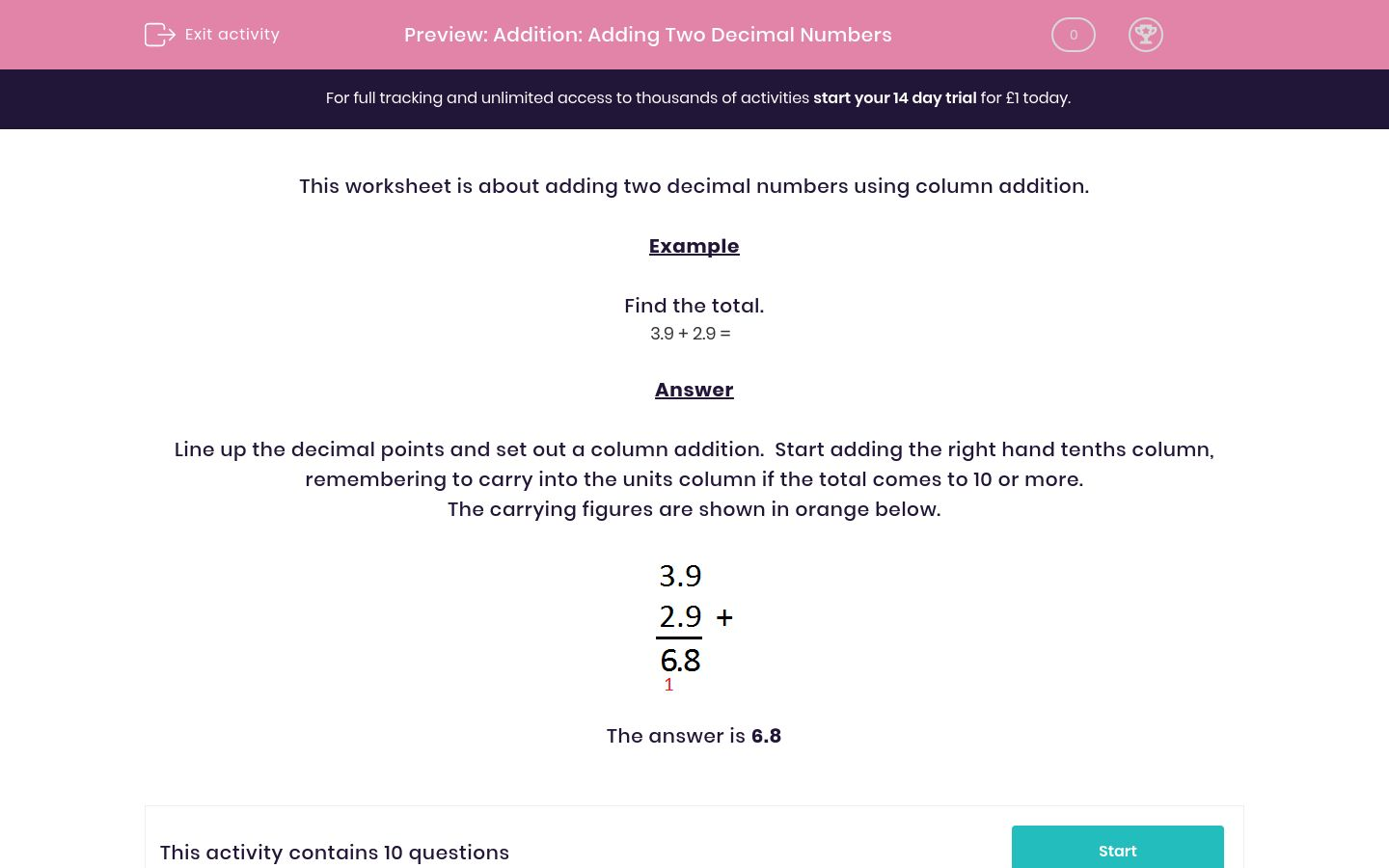### Affordable pricing from £10/monthKey stage:  KS 2

Curriculum topic:   Maths and Numerical Reasoning

Curriculum subtopic:   Decimals

Difficulty level:### QUESTION 1 of 10

Example

Find the total.

 3.9 + 2.9 =

Line up the decimal points and set out a column addition.  Start adding the right hand tenths column, remembering to carry into the units column if the total comes to 10 or more.

The carrying figures are shown in orange below.Find out the total.

8.8 + 3.2 =

Find out the total.

0.7 + 5.4 =

Find out the total.

6.8 + 5.9 =

Find out the total.

4.6 + 1.5 =

Find out the total.

2.1 + 3.2 =

Find out the total.

2.8 + 7 =

Find out the total.

4.5 + 7 =

Find out the total.

3.4 + 2.5 =

Find out the total.

3 + 2.3 =

Find out the total.

7.8 + 1.2 =
• Question 1

Find out the total.

8.8 + 3.2 =
12
• Question 2

Find out the total.

0.7 + 5.4 =
6.1
• Question 3

Find out the total.

6.8 + 5.9 =
12.7
• Question 4

Find out the total.

4.6 + 1.5 =
6.1
• Question 5

Find out the total.

2.1 + 3.2 =
5.3
• Question 6

Find out the total.

2.8 + 7 =
9.8
• Question 7

Find out the total.

4.5 + 7 =
11.5
• Question 8

Find out the total.

3.4 + 2.5 =
5.9
• Question 9

Find out the total.

3 + 2.3 =
5.3
• Question 10

Find out the total.

7.8 + 1.2 =
9
---- OR ----

Sign up for a £1 trial so you can track and measure your child's progress on this activity.

### What is EdPlace?

We're your National Curriculum aligned online education content provider helping each child succeed in English, maths and science from year 1 to GCSE. With an EdPlace account you’ll be able to track and measure progress, helping each child achieve their best. We build confidence and attainment by personalising each child’s learning at a level that suits them.

Get started